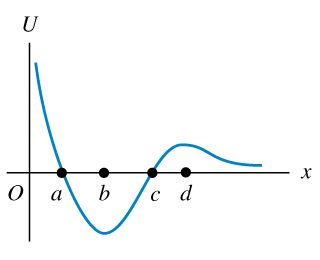# Problem: A marble moves along the x-axis. The potential-energy function is shown in the figure .At which of the labeled x-coordinates is the force on the marble zero?Which of the labeled x-coordinates is a position of stable equilibrium?Which of the labeled x-coordinates is a position of unstable equilibrium?

⚠️Our tutors found the solution shown to be helpful for the problem you're searching for. We don't have the exact solution yet.

###### Problem Details

A marble moves along the x-axis. The potential-energy function is shown in the figure.

At which of the labeled x-coordinates is the force on the marble zero?

Which of the labeled x-coordinates is a position of stable equilibrium?

Which of the labeled x-coordinates is a position of unstable equilibrium?